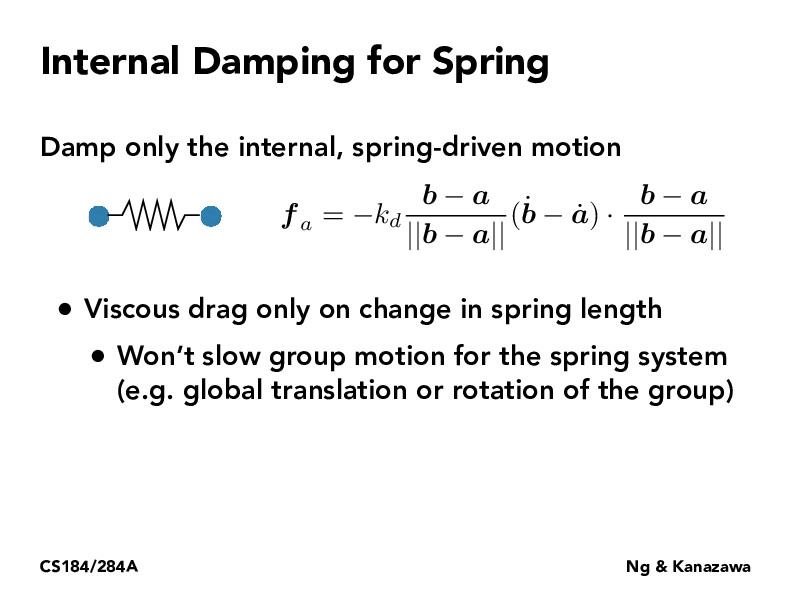Lecture 18: Introduction to Physical Simulation (15)herojelly

Why are there two $\frac{b-a}{||b-a||}$ terms?briana-jin-zhang

The first one is used to normalize the direction and the second one is so you do a dot product aka projection in order to find out how much in that particular direction.Leo-godel

I am curious about why here there are two (b-a)/|b-a| terms. Since for the result, these two terms multiply will become constant 1.

You must be enrolled in the course to comment